Click to Chat

1800-1023-196

+91-120-4616500

CART 0

• 0

MY CART (5)

Use Coupon: CART20 and get 20% off on all online Study Material

ITEM
DETAILS
MRP
DISCOUNT
FINAL PRICE
Total Price: Rs.

There are no items in this cart.
Continue Shopping• Complete Physics Course - Class 11
• OFFERED PRICE: Rs. 2,968
• View Details

Introduction to Motion in One Dimension

Table of Content

Motion in One Dimension

Frame of Reference

Position of an Object

Position Vector

Displacement

Difference Between Distance and Displacement

Uniform and Non Uniform Motion

Graphical Representation of the Uniform Motion

Velocity Vector in Non Uniform Motion

Instantaneous velocity

Acceleration

Acceleration Vector in Non Uniform Motion

Variable Acceleration

Equations of Motion

Related Resources

A body

A certain amount of matter limited in all directions and consequently having a finite size, shape and occupying some definite space is called a body.

Particle

A particle is defined as a portion of matter infinitesimally small in size so that for the purpose of investigation, the distance between its different parts may be neglected. Thus, a particle has only a definite position, but no dimension. In the problems we are going to discuss, we will consider a body to be a particle for the sake of simplicity.

MotionThe position of object can change on a straight line (like on x-axis with respect to origin) or on a plane with respect to some fixed point on frame. So we can define motion as follows:-

An object or a body is said to be in motion if its position continuously changes with time with reference to a fixed point (or fixed frame of reference).

But note that, the moving object is either a particle, a point object (such as an electron) or an object that moves like a particle. A body is said to be moving like if every portion of it moves in the same direction and at the same rate.

Motion in One Dimension

When the position of object changes on a straight line i.e. motion of object along straight line is called motion in one dimension.

To understand the essential concepts of one dimensional motion we have to go through some basic definitions.

Frame of Reference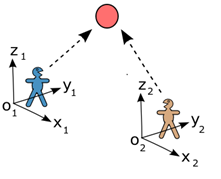One can see the platform from a running train, and it seems that all the objects placed on platform are continuously changing their position. But one, who is on platform, concludes that the objects on the platform are at rest. It means if we will take the trains are reference frame the objects are not stationary and taking reference frame as platform the objects are stationary. So the study of motion is a combined property of the object under study and the observer. Hence there is a need to define a frame of reference under which we have to study the motion of an object.

Definition

A frame of reference is a set of coordinate axes which is fixed with respect to a space point (a body or an object can also be treated as a point mass therefore it can become a site for fixing a reference frame), which we have arbitrarily chosen as per our observer's requirement. The essential requirement for a frame of reference is that, it should be rigid.

Position of an Object

The position of an object is defined with respect to some frame of reference. As a convention, we define position of a point (essentially we treat body as a point mass) with the help of three co-ordinates X, Y and Z. Hence X, Y, Z is a set of coordinate axes representing a 3-dimensional space and each point in this space can be uniquely defined with the help of a set of X, Y and Z coordinate, all three axes being mutually perpendicular to each other. The line drawn from origin to the point represents the position vector of that point.

Position Vector

It describes the instantaneous position of a particle with respect to the chosen frame of reference. It is a vector joining the origin to the particle. If at any time, (x, y, z) be the Cartesian coordinates of the particle then its position vector is given by vector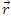= xi + yj + zk.

In one-dimensional motion: vector= xi,     y = z = 0   (along x-axis)

In two-dimensional motion: vector= xi + yj                 (in x-y plane z = 0)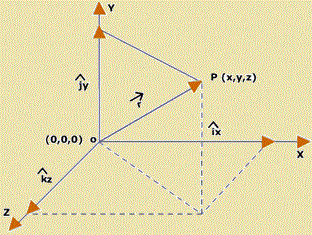In the figure above, the position of a point P is specified and vector OP is called the position vector.

Displacement

Consider a case in which the position of an object changes with time. Suppose at certain instant 't' the position of an object is x1 along the x axis and some other instant 'T' the position is x1 then the displacement Δx is defined as,

Δx = x2 - x1It can be seen in the figure above where x1 and x2 are instantaneous position of the object at that time.Now consider the motion of a point A with respect to a reference point O. The motion of point A makes its radius vector vary in the general case both in magnitude and in direction as shown in figure above. Suppose the point A travels from point 1 to point 2 in the time interval Δt. It is seen from the figure that the displacement vector Δof the point A represents the increment of vectorin time Δt:

Δ=2 -t

Difference Between Distance and Displacement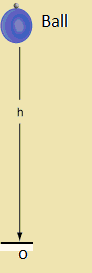To understand the difference between distance and displacement, we study the motion of vertical throw of a ball with respect to point O, as shown in the left figure, to height h.

After some time it will come again to the same point O. The displacement of ball is zero but there is some distance traversed by the ball. It's because distance is a scalar quantity but displacement is a vector quantity.

Uniform and Non Uniform MotionSpeed is the rate of change of distance without regard to directions. Velocity is the rate at which the position vector of a particle changes with time. Velocity is a vector quantity whereas speed is scalar quantity but both are measured in the same unit m/sec.

The motion of an object may be uniform or non-uniform depending upon its speed. In case of uniform motion the speed is constant, whereas in the non-uniform motion, the speed is variable.

In uniform motion in one dimension the velocity (v) is mathematically defined as

v = (x2 - x1)/(T-t)     ...... (1)

Where x1 and x2 are instantaneous displacement as shown in figure above at time 't' and 'T' respectively.

Graphical Representation of the Uniform Motion

Form the equation (1) we have the following equation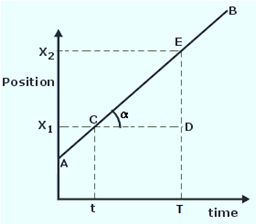x2 = x1 + v(T - t)

where v is constant. Take t = 0, the equation becomes x2 = x1 + vT, from this equation it follows that the graph of position of object 'x2' against 'T' is a straight line, cutting off x1 on the position axis where x1 is the distance of the particle from the origin at time t = 0.

v = slope of the graph which is constantNote:-

If the graph is not a straight line, it will represent non-uniform motion.

Motion of a body cannot be correctly identified unless one knows the position of body as specified by a fixed frame of reference.

Velocity Vector in Non Uniform Motion

In any non-uniform motion, we can define an average velocity over a time interval. Average velocity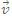is the ratio of the displacement Δx (that occurs during a particle time interval Δt) to that interval of time i.e.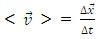Now refer to the example, related to figure 2.3, the ratio of Δ/Δt is called the average velocity <> during the time interval Δt. The direction of the vector <> coincides with that of Δ. Average velocity is also a vector quantity.

Note:  The ratio of total distance traveled and time taken during the motion is called average speed. Average speed is a scalar quantity.

If at any time t1 position vector of the particle is1 and at time2 position vector is2 then for this interval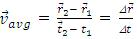Instantaneous velocity

Instantaneous velocity is defined as the rate of change of displacement.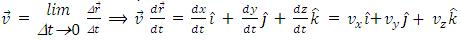Velocity

The velocity at any instant is obtained from the average velocity shrinking the time interval closer to zero. As Δt tends to zero, the average velocity approaches a limiting value, which is the velocity at that instant, called instantaneous velocity, which is a vector quantity, mathematically we can define it as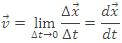The magnitude v of the instantaneous velocity is called the speed and is simple the absolute value ofIn the example related with figure given below, the instantaneous velocity is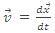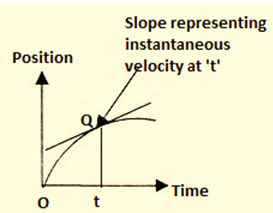Hence instantaneous velocity is the rate at which a particle's position is changing with respect to time at a given instant. The velocity of a particle at any instant is the slope (tangent) of its position curve at the point representing that instant of time, as shown in figure above.

Speed

Speed is defined as rate of change of distance with time.

In any interval of time, average speed is defined as

<speed> = (total distance)/(total time taken) = Δs/Δt. As Δs > |Δ|, hence <speed> > <velocity>

Think :   (i)Can a body have a constant speed and still have a varying velocity?
(ii)Can a body have a constant velocity and still have a varying speed?

Problem 1:-

This question contains statement-1 (Assertion) and Statement-2 (Reason). Question has 4 choices (A), (B), (C) and (D) out of which only one is correct.

Statement-1

A bus moving due north take a turn and starts moving towards east with same speed. There will be no change in the velocity of the bus.

Statement-2

Velocity is a vector quantity.

(A)  Statement-1 is true, Statement-2 is true, Statement-2 is a correct explanation for statement-1.

(B)  Statement-1 is true, Statement-2 is true, Statement-2 is not a correct explanation for statement-1.

(C)  Statement-1 is true, Statement-2 is false.

(D)  Statement-1 is false, Statement-2 is true.

Solution:- (D)

This is so because bus is changing its direction of motion.

______________________________________________________________________________________________

Problem 2:-

A man started running form origin and went up to (2, 0) and returned back to (-1, 0) as shown in figure 2.7. In this process total time taken by man is 2 seconds. Find the average velocity and average speed.Solution:-

The man is displaced form origin to (-1, 0)

Hence displacement, s =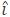So average velocity  = Displacement/total time =/2 = (-1/2)m/sec.

where as, since the total distance traveled by man

= (0, 0) to (2, 0)+(2, 0) to (0, 0)+(0, 0) to (-1, 0)

= 2 + 2 + 1 = 5 m

Hence average speed

= (Total distance)/(Total time)=5/2 m/sec.

______________________________________________________________________________________________

Probem 3:-

A cyclist moves 12 km due to north and then 5 km due east in 3 hr. Find (a) his average speed, (b) average velocity, in m/s.

Solution:-

In the figure, A shows the initial position and C the final position of the cyclist. The total distance covered by the cyclist AB+BC= (12+5)km = 17 km.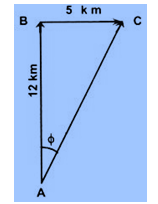So, its average speed = 17/3 km/hr = 1.57 m/s

Its displacement is AC and the magnitude is given by

AC = √(AB2 + BC2) = √(122 + 52) km = 13 km

Thus, its average velocity = 13/3 km/hr

= 1.2 m/s along AC, i.e at tan-1(5/12) or 22.6o East of North.

_______________________________________________________________________________________________

Problem 4:-

A train is moving with a constant speed of 5 m/s and there are two persons A and B standing at a separation of 10 m inside the train. Another person C is standing on the ground. Then, find

(a)    displacement covered by A, if he moves towards B and back to its position in 10 seconds in frame of reference of train and in frame of reference of C.

(b)    distance covered by A in frame of reference of train and in frame of reference of C.

Solution:-

(a)    In the frame of train, displacement covered by A is zero and in frame of reference of C, displacement covered by A=0 + 5x10 = 50 m.

(b)    Distance covered by A in frame of reference of train is 20m and distance covered by A in frame of reference of C is (20 + 50) = 70 m.The dispalcement remains unaffected due to shifting of origin from one point to the other.

The displacement can have positive, negative or zero value.

The dispalcement is never greater than the actual distance travelled.

The displacement has unit of length.

Velocity can be considered to be a combination of speed and direction.

A change in either speed or direction of motion results in a change in velocity.

It is not possible for a particle to possess zero speed with a non-zero velocity.

A particle which completes one revolution, along a circular path, with uniform speed is said to possesss zero velocity and non-zero speed.

In case a body moves with uniform velocity, along a straight line, its average speed is equal to its instantaneous speed.

Acceleration

Acceleration is the rate of change of velocity with time. The concept of acceleration is understood in non-uniform motion. It is a vector quantity.

Average acceleration is the change in velocity per unit time over an interval of time.

Instantaneous acceleration is defined as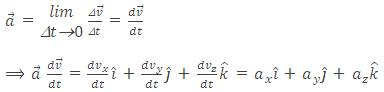Acceleration Vector in Non Uniform MotionSuppose that at the instant t1 a particle as in figure above, has velocity  and at t2, velocity is . The average acceleration during the motion is defined as

Variable Acceleration

The acceleration at any instant is obtained from the average acceleration by shrinking the time interval closer zero. As Δt tends to zero average acceleration approaching a limiting value, which is the acceleration at that instant called instantaneous acceleration which is vector quantity.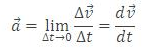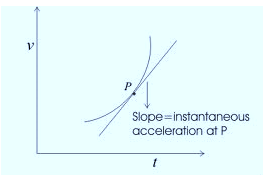i.e. the instantaneous acceleration is the derivative of velocity.

Hence instantaneous acceleration of a particle at any instant is the rate at which its velocity is changing at that instant. Instantaneous acceleration at any point is the slope of the curve v (t) at that point as shown in figure above.

Equations of Motion

The relationship among different parameter like displacement velocity, acceleration can be derived using the concept of average acceleration and concept of average acceleration and instantaneous acceleration.

When acceleration is constant, a distinction between average acceleration and instantaneous acceleration loses its meaning, so we can write

where  is the velocity at t = 0 and  is the velocity at some time t.

So,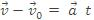.................. (2)

This is the first useful equation of motion.

Similarly for displacement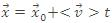.................. (3)

in which  is the position of the particle at t0 and  is the average velocity between t0 and later time t. If at t0 and t the velocity of particle is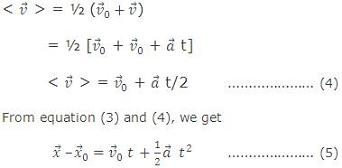This is the second important equation of motion.

Now from equation (2), square both side of this equation we getThis is another important equation of motion.Note:

The equation of motion derived above are possible only in uniformly accelerated motion i.e. the motion in which the acceleration is constant.

Refer this video for better understanding about motion in one dimension:-

Problem 5:-

The nucleus of helium atom (alpha-particle) travels inside a straight hollow tube of length 2.0 meters long which forms part of a particle accelerator. (a) If one assumes uniform acceleration, how long is the particle in the tube if it enters at a speed of 1000 meter/sec and leaves at 9000 meter/sec? (b) What is its acceleration during this interval?

Solution:-

(a) We choose x-axis parallel to the tube, its positive direction being that in which the particle is moving and its origin at the tube entrance. We are given x and vx and we seek t. The acceleration ax is not involved. Hence we use equation 3, x = x0 + <v> t.

We get,

x = v0 + ½ (vx0) + vx) t, with x0 = 0 or

t = 2x/(vx0+vx),

t = ((2)(2.0 meters))/((1000+9000)meters/sec) = 4.0/10-4 sec    Ans.

(b) The acceleration follows from equation 2, vx = vx0 + axt

=> ax = (v0-vx0)/t = ((9000-1000)meters/sec)/(4.0×10(-4) sec)

= 2.0 × 107 meter/sec2 Ans.

The above equations of motion are, however, universal and can be derived by using differential calculus as given below: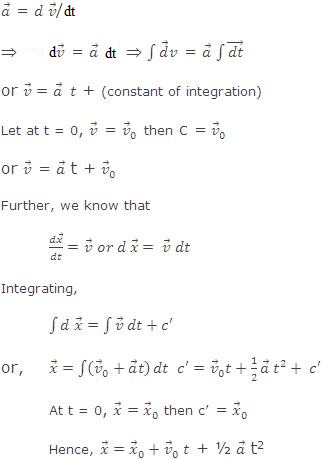Thus, we have derived the same equation of motion using calculus.

To understand the use of calculus in solving the kinematics problems we can look into the following illustrations.

__________________________________________________________________________________________________

Problem 6:-

The displacement x of a particle moving in one dimension, under the action of a constant force is related to the time t by the equation t = √x + 3 where x is in meter and t is in seconds. Find the displacement of the particle when its velocity is zero.

Solution:-

Here t = √x + 3 => √x = t - 3

Squaring both sides, we get x = t2 - 6t + 9,

As we know velocity, v = dx/dt

Hence we get v = dx/dt = 2t - 6

Put v = 0, we get, 2t - 6 = 0

So,t = 3s

When t = 3s, x = t2 - 6t + 9 = 9 - 6(3) + 9 = 0

Hence the displacement of the particle is zero when its velocity is zero.

_________________________________________________________________________________________________

Problem 7:-

A particle starts from a point whose initial velocity is v1 and it reaches with final velocity v2, at point B which is at a distance 'd' from point A. The path is straight line. If acceleration is proportional to velocity, find the time taken by particle from A to B.

Solution:-

Here acceleration a is proportional to velocity v.

Hence a α v

=> a = kv, where k is constant

=> dv/dt = kv ............... (1)

=> (dv/ds)(ds/dt) = kv => (dv/ds) v = kv

=> k = (v2-v1)/d

From equation (1)

=> (dv/v)= k(dt)

or, ln (v2/v1) = kt

So, t =  [ln (v2/v1)]/k = [d/(v2-v1)][ln (v2/v1)]Question 1:-

What determines the nature of path following by the particle?

(a) speed         (b) velocity       (c) acceleration     (d) none of these

Querstion 2:-

A moving body is covering distances in proportion to the square of time along a straight line. The acceleration of the body is:

(a) increasing    (b) decreasing   (c) zero     (d) constant

Querstion 3:-

The distance covered by a body in time ‘t’ is propertional to the square of the time ‘t’. The acceleration of the body is:

(a) zero     (b) constant        (c) increasing       (d) decreasing

Querstion 4:-

A train travels 4 km due east and then 3 km due north and finally comes back to the starting position travelling 5 km along south-west direction. What is the net displacement?

(a) 12 km    (b) 5 km   (c) zero    (d) 1 km

Querstion 5:-

A body moves along a straight line, the motion of the body is said to be:

(a) one dimensional              (b) two dimensional

(c) three dimensional           (d) may be any of these three.Q.1

Q.2

Q.3

Q.4

Q.5

a

d

b

c

d

Related Resources:-

You might like to refer Kinematics.

For getting an idea of the type of questions asked, refer the  Previous Year Question Papers.

To read more, Buy study materials of Kinematics comprising study notes, revision notes, video lectures, previous year solved questions etc. Also browse for more study materials on Physics here.### Course Features

• 101 Video Lectures
• Revision Notes
• Previous Year Papers
• Mind Map
• Study Planner
• NCERT Solutions
• Discussion Forum
• Test paper with Video Solution